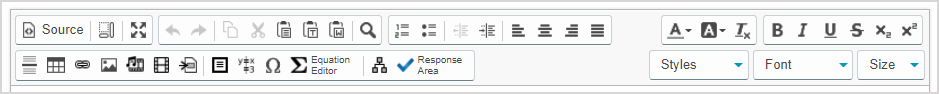# View available question types

A range of question types are possible with Möbius.The question type is defined using the Response Area iconfrom the Visual Editor (contained within the Question Editor).TIP: A single question can have multiple response areas.

IMPORTANT: If you don't interact with Möbius for 60 minutes, you'll be automatically logged out of your session and your progress isn't auto-saved. If you plan to stop interacting with the Question Editor for more than a few minutes, it's strongly recommended that you save your work.

TIP: Check out Use the Visual Editor for details on how to use this toolbar to the fullest to control the visual appearance of your question text while authoring your question.TIP: You can also author an adaptive question which is a specialized question type. Adaptive questions consist of multiple sections that are presented to a student one at a time to moderate the student's progression through a specific question. Each section can contain any number and combination of response areas. Check out Author an adaptive question for full authoring details.

TIP: Most question types can be authored with algorithmic variables to become algorithmic questions. Check out Author an algorithmic question for help with transforming a static question into an algorithmic question.

## Clickable image

A clickable image question presents an image with a customizable number of clickable hot spots.

Students are required to identify the correct image element by clicking the appropriate hot spot.

Check out Author a clickable image question.

A document upload question allows students to upload a document during an activity or generates a unique code that can be used by the instructor to upload a document later on the student's behalf.

The document to be uploaded could be anything you want to collect to support a specific question. Example — An offline portion of an exam or a photo of a student's scratch paper that accompanies a certain question.

Check out Author a document upload question.

## Essay

An essay question is typically used for a long answer response. Example — Mathematical proofs, long answer questions, or essays.

Check out Author an essay question.

## Free body diagram

A free body diagram question can be used to ask a student to draw forces from one or more starting points—or control points—over a background image.

Check out Author a free body diagram question.

## HTML

An HTML question provides Javascript hooks that allow an author to connect web applications to the grading system.

Both existing and purpose-built HTML + Javascript applications may be used as questions if they can be appropriately configured.

Check out Author an HTML question.

## List

A list question offers a fill-in-the-blank style response using a drop-down list or free text entry.

Multiple correct or incorrect responses can be defined with partial credits for each response.

Check out Author a list question.

A Maple-graded question includes facilities for algebra, calculus, differential equations, discrete mathematics, graphics, numerical computation, and many other areas of mathematics.

This question type gives you access to the computational power and plotting capabilities of the MapleTM math engine.

Additionally, you can also use functions and routines that are contained in a separate Maple Repository (Maple Library) by accessing your personal Maple library archives from within your Maple questions.

Check out Author a Maple-graded question.

NOTE: There are two Maple-graded sub-types that can be authored:

• Formula
• Maple syntax

## Matching

A matching question displays two lists.

The student must match each element of the first list with an element of the second list.

Check out Author a matching question.

## Math App

A Math App question contains an embedded Maple worksheet that the student can interact with.

The worksheet will be graded in a final state that the student leaves it in.

Check out Author a Math App question.

## Mathematical formula

A mathematical formula question allows instructors to compare a student response—usually a mathematical expression or equation—to a specified answer ( Example — symbolic expression, algebraic expression, equation expression, or chemical equation).

Check out Author a mathematical formula question.

NOTE: There are ten mathematical formula sub-types that can be authored:

• Formula
• Formula without logs and trig
• Formula with physical units
• Formula that matches responses to within +C
• Formula without simplification
• Equation
• Unordered list of formulas
• Ordered lists of formulas
• Vectors of formulas
• Chemical equation

Check out Choose a mathematical formula question sub-type for more information on each available sub-type.

## Multiple choice

A multiple choice question can have any number of choices with exactly one correct answer or require students to select more than one answer.

The answer choices can be presented in fixed or random order.

Answer choices can be displayed horizontally or vertically.

Check out Author a multiple choice question.

## Numeric

A numeric question compares a student's response to a given number that can contain a unit of measure.

You can set the grading accuracy and allowed tolerance of a student's response compared to the correct answer.

Check out Author a numeric question.

## Sketch

A sketch question is used to assess a student's ability to plot and interact with a variety of different lines and curves.

Check out Author a sketch question.

## Sorting

A sorting question allows the student to click-and-drag sortable items to re-arrange them into the a correct order (Examplesmallest to largest, shortest to longest, etc.).

Check out Author a sorting question.

## True or false

A true or false question allows you to ask a modified version of a multiple choice question with only two answer choices: True and False.

Check out Author a true or false question.